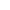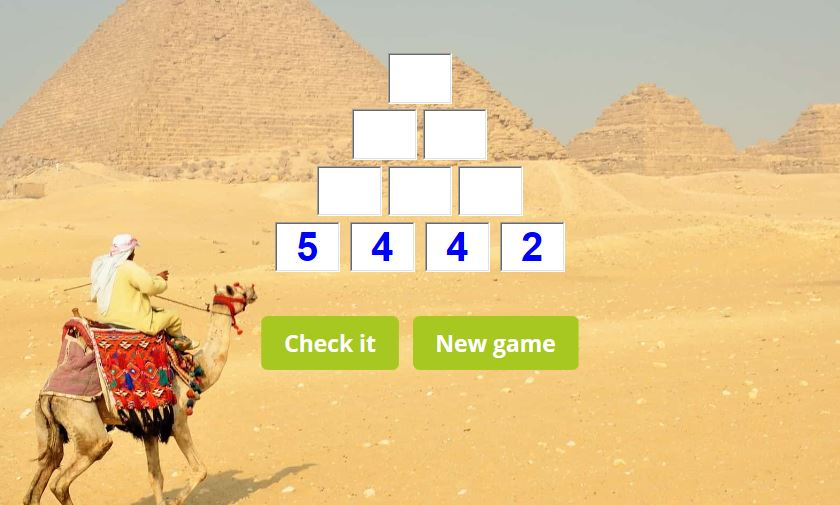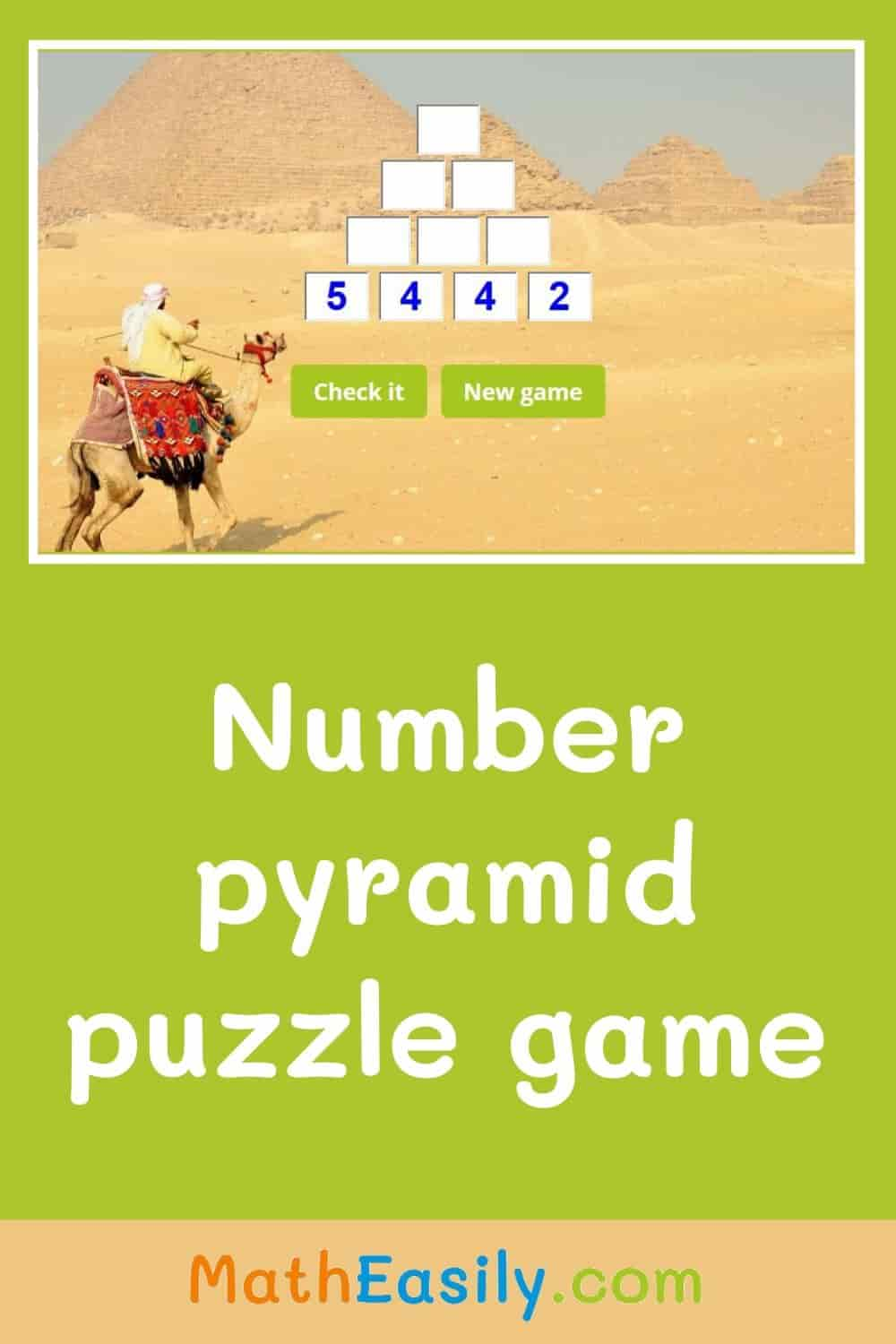﻿ Math Number Pyramid Puzzle Game + Worksheets

Page No. 1016

# Number pyramid puzzle game

Complete the addition pyramid puzzle. Solve math number pyramid puzzle game online. Play missing number pyramid puzzle for kids. Learn how to solve number pyramids. Download math pyramid puzzle worksheet, number pyramids worksheet, addition pyramids worksheet and 4-layer number pyramid online. Play math number pyramid game. Learn how to play math pyramid game. number pyramid generator. Download printable math pyramid worksheet and pyramid addition worksheets. Play number pyramid game.

New gameFill in missing numbers in the number pyramid puzzle game.
Click the blue button to choose the maximum value on the top: 20, 30, 50 or 100:

## Math number pyramid puzzle game onlineMath number pyramids are pyramids of numbers. In a number pyramid puzzle game, numbers next to each other are added together to make the number above it.

You can choose the maximum value on the top of the addition pyramid: 20, 30, 50 or 100. Math pyramid puzzle game is a great way how kids can learn addition.Addition pyramids worksheets help you to make math lessons more interesting. Kids have to complete the missing number pyramid puzzle.

Download our free printable addition pyramids worksheets in PDF below. Each number pyramids worksheet consists of two pages: the first page is the worksheet, the other page contains the answer key. All these number pyramid puzzles are generated automatically: every time a new addition pyramid worksheet.

You can find even more free 1st grade math worksheets here.

## How do you solve an addition pyramid math puzzle?

In the addition pyramid math puzzle kids must add together two numbers. They can use the knowledge they have gained from playing the number bonds game.

Students solve the math pyramid game by adding adjacent numbers and writing the sum above them, working this way up to the top of the 4-layer number pyramid puzzle.

The bottom row is filled with random numbers. Each number in the upper rows is the sum of the two numbers below it.

Press "Check it" to find out if your answer is OK. The correct boxes will be green, wrong boxes will be red. Press the button "New game" and you can play a new number pyramid puzzle game. You can also watch this tutorial on YouTube.

## Why to play math pyramid puzzle game?

Number pyramid game is a nice way how to learn addition. To play this game kids need to have basic knowledge of addition up to 20 and addition up to 30.

Math pyramid puzzle game is suitable for training 1st Grade Math. Do you want to practice addition and subtraction at the same time? Then play this math crossword puzzle game.

### You might also like these number pyramid games:## Pyramid number puzzle

In math pyramid puzzle game every brick contains the sum of the two bricks below. Fill in the missing numbers in the empty bricks.

This pyramid number puzzle can be played on any device, you need only the internet connection.